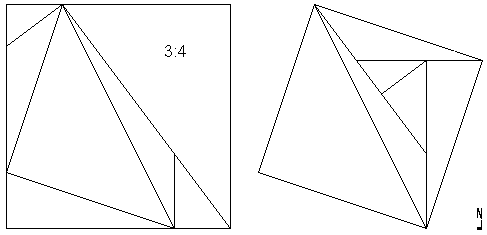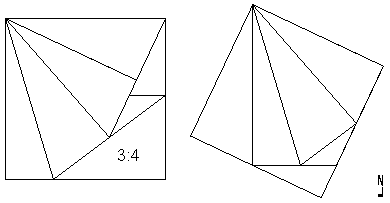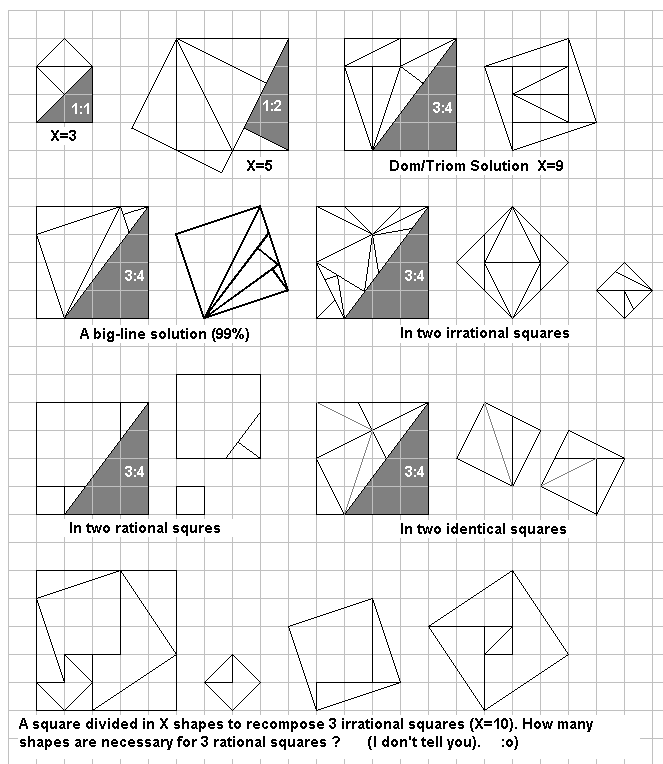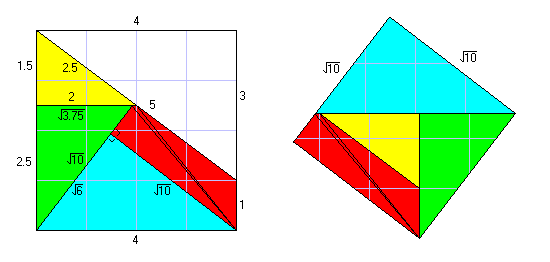Wei-Hwa Huang's Challenge

Wei-Hwa Huang sent this puzzle.  "Divide a square into 7 triangles.  One triangle must have edge lengths in the ratio 3:4:5.  The other 6 triangles must be arrangeable into another square!"  He asks if the same task can be done with less than seven triangles.

Wei-Hwa Huang's Pythagorean challenge has at least 4 solutions with 7 triangles.  These were found by Wei-Hwa Huang, Junk Kato, Andrew Cook, and Livio Zucca.Joseph DeVincentis and Livio Zucca found a nine triangle solution.  See the Dom/Triom Solution below.Solution by Andrew Cook (and Junk Kato)